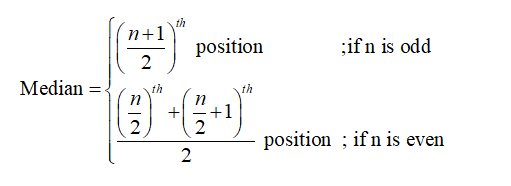Question
1 views

How to find measures of center and measures of spread?

check_circle

Step 1

The measures of center are mean, median and mode.

Mean:

Mean is a measure of center in case of quantitative data. Mean of a data set is the sum of the data set divided by the size.

The general formula for mean is,

X bar=sum of observations xi/total number of observations n

Step 2

Median:

The median is the middle value in an ordered data set. If there are no ties, half of the observations will be smaller than the median, and half of the observations will be larger than the median.

The formula for median is as follows:Mode:

The most repeated value in the data set is termed as mode.

&...

### Want to see the full answer?

See Solution

#### Want to see this answer and more?

Solutions are written by subject experts who are available 24/7. Questions are typically answered within 1 hour.*

See Solution
*Response times may vary by subject and question.
Tagged in

### Statistics